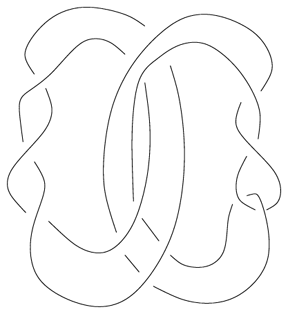# Jones unknotting conjecture

Every non-trivial knot has a non-trivial Jones polynomial.Figure: j130050a

The conjecture has been confirmed for several families of knots, including alternating and adequate knots, knots up to \$18\$ crossings and \$2\$-algebraic knots (cf. Knot theory) up to \$21\$ crossings [a5], [a7]. Recently (2001), S. Yamada announced that the conjecture holds for knots with up to \$20\$ crossings. The analogous conjecture for links does not hold, as M.B. Thistlethwaite found a \$15\$-crossing link whose Jones polynomial coincides with a trivial link of two components, cf. Fig.a1. This and similar examples constructed since are \$2\$-satellites on a Hopf link [a6], [a1].

L.H. Kauffman showed that there are non-trivial virtual knots with Jones polynomial equal to \$1\$, [a4].

It is still an open problem (as of 2001) whether a simple (non-satellite) link can have a Jones polynomial of an unlink.

How to Cite This Entry:
Jones unknotting conjecture. Encyclopedia of Mathematics. URL: http://encyclopediaofmath.org/index.php?title=Jones_unknotting_conjecture&oldid=39362
This article was adapted from an original article by Jozef Przytycki (originator), which appeared in Encyclopedia of Mathematics - ISBN 1402006098. See original article